# Title 40

## SECTION 53.53

### 53.53 Test for flow rate accuracy, regulation, measurement accuracy, and cut-off.

§ 53.53 Test for flow rate accuracy, regulation, measurement accuracy, and cut-off.

(a) Overview. This test procedure is designed to evaluate a candidate sampler's flow rate accuracy with respect to the design flow rate, flow rate regulation, flow rate measurement accuracy, coefficient of variability measurement accuracy, and the flow rate cut-off function. The tests for the first four parameters shall be conducted over a 6-hour time period during which reference flow measurements are made at intervals not to exceed 5 minutes. The flow rate cut-off test, conducted separately, is intended to verify that the sampler carries out the required automatic sample flow rate cut-off function properly in the event of a low-flow condition. The test conditions and performance specifications associated with this test are summarized in table E-1 of this subpart. The candidate test sampler must meet all test parameters and test specifications to successfully pass this test.

(b) Technical definitions. (1) Sample flow rate means the quantitative volumetric flow rate of the air stream caused by the sampler to enter the sampler inlet and pass through the sample filter, measured in actual volume units at the temperature and pressure of the air as it enters the inlet.

(2) The flow rate cut-off function requires the sampler to automatically stop sample flow and terminate the current sample collection if the sample flow rate deviates by more than the variation limits specified in table E-1 of this subpart (±10 percent from the nominal sample flow rate) for more than 60 seconds during a sample collection period. The sampler is also required to properly notify the operator with a flag warning indication of the out-of-specification flow rate condition and if the flow rate cut-off results in an elapsed sample collection time of less than 23 hours.

(c) Required test equipment. (1) Flow rate meter, suitable for measuring and recording the actual volumetric sample flow rate at the sampler downtube, with a minimum range of 10 to 25 L/min, 2 percent certified, NIST-traceable accuracy. Optional capability for continuous (analog) recording capability or digital recording at intervals not to exceed 30 seconds is recommended. While a flow meter which provides a direct indication of volumetric flow rate is preferred for this test, an alternative certified flow measurement device may be used as long as appropriate volumetric flow rate corrections are made based on measurements of actual ambient temperature and pressure conditions.

(2) Ambient air temperature sensor, with a resolution of 0.1 °C and certified to be accurate to within 0.5 °C (if needed). If the certified flow meter does not provide direct volumetric flow rate readings, ambient air temperature measurements must be made using continuous (analog) recording capability or digital recording at intervals not to exceed 5 minutes.

(3) Barometer, range 600 mm Hg to 800 mm Hg, certified accurate to 2 mm Hg (if needed). If the certified flow meter does not provide direct volumetric flow rate readings, ambient pressure measurements must be made using continuous (analog) recording capability or digital recording at intervals not to exceed 5 minutes.

(4) Flow measurement adaptor (40 CFR part 50, appendix L, figure L-30) or equivalent adaptor to facilitate measurement of sample flow rate at the sampler downtube.

(5) Valve or other means to restrict or reduce the sample flow rate to a value at least 10 percent below the design flow rate (16.67 L/min). If appropriate, the valve of the flow measurement adaptor may be used for this purpose.

(6) Means for creating an additional pressure drop of 55 mm Hg in the sampler to simulate a heavily loaded filter, such as an orifice or flow restrictive plate installed in the filter holder or a valve or other flow restrictor temporarily installed in the flow path near the filter.

(7) Teflon sample filter, as specified in section 6 of 40 CFR part 50, appendix L (if required).

(d) Calibration of test measurement instruments. Submit documentation showing evidence of appropriately recent calibration, certification of calibration accuracy, and NIST-traceability (if required) of all measurement instruments used in the tests. The accuracy of flow-rate meters shall be verified at the highest and lowest pressures and temperatures used in the tests and shall be checked at zero and at least one flow rate within ±3 percent of 16.7 L/min within 7 days prior to use for this test. Where an instrument's measurements are to be recorded with an analog recording device, the accuracy of the entire instrument-recorder system shall be calibrated or verified.

(e) Test setup. (1) Setup of the sampler shall be as required in this paragraph (e) and otherwise as described in the sampler's operation or instruction manual referred to in § 53.4(b)(3). The sampler shall be installed upright and set up in its normal configuration for collecting PM samples. A sample filter and (or) the device for creating an additional 55 mm Hg pressure drop shall be installed for the duration of these tests. The sampler's ambient temperature, ambient pressure, and flow rate measurement systems shall all be calibrated per the sampler's operation or instruction manual within 7 days prior to this test.

(2) The inlet of the candidate sampler shall be removed and the flow measurement adaptor installed on the sampler's downtube. A leak check as described in the sampler's operation or instruction manual shall be conducted and must be properly passed before other tests are carried out.

(3) The inlet of the flow measurement adaptor shall be connected to the outlet of the flow rate meter.

(4) For the flow rate cut-off test, the valve or means for reducing sampler flow rate shall be installed between the flow measurement adaptor and the downtube or in another location within the sampler such that the sampler flow rate can be manually restricted during the test.

(f) Procedure. (1) Set up the sampler as specified in paragraph (e) of this section and otherwise prepare the sampler for normal sample collection operation as directed in the sampler's operation or instruction manual. Set the sampler to automatically start a 6-hour sample collection period at a convenient time.

(2) During the 6-hour operational flow rate portion of the test, measure and record the sample flow rate with the flow rate meter at intervals not to exceed 5 minutes. If ambient temperature and pressure corrections are necessary to calculate volumetric flow rate, ambient temperature and pressure shall be measured at the same frequency as that of the certified flow rate measurements. Note and record the actual start and stop times for the 6-hour flow rate test period.

(3) Following completion of the 6-hour flow rate test period, install the flow rate reduction device and change the sampler flow rate recording frequency to intervals of not more than 30 seconds. Reset the sampler to start a new sample collection period. Manually restrict the sampler flow rate such that the sampler flow rate is decreased slowly over several minutes to a flow rate slightly less than the flow rate cut-off value (15.0 L/min). Maintain this flow rate for at least 2.0 minutes or until the sampler stops the sample flow automatically. Manually terminate the sample period, if the sampler has not terminated it automatically.

(g) Test results. At the completion of the test, validate the test conditions and determine the test results as follows:

(1) Mean sample flow rate. (i) From the certified measurements (Qref) of the test sampler flow rate obtained by use of the flow rate meter, tabulate each flow rate measurement in units of L/min. If ambient temperature and pressure corrections are necessary to calculate volumetric flow rate, each measured flow rate shall be corrected using its corresponding temperature and pressure measurement values. Calculate the mean flow rate for the sample period (Qref,ave) as follows:

Equation 1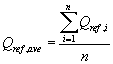where: n equals the number of discrete certified flow rate measurements over the 6-hour test period.

(ii)(A) Calculate the percent difference between this mean flow rate value and the design value of 16.67 L/min, as follows:

Equation 2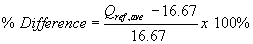(B) To successfully pass the mean flow rate test, the percent difference calculated in Equation 2 of this paragraph (g)(1)(ii) must be within ±5 percent.

(2) Sample flow rate regulation. (i) From the certified measurements of the test sampler flow rate, calculate the sample coefficient of variation (CV) of the discrete measurements as follows:

Equation 3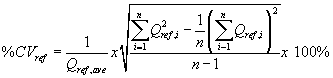(ii) To successfully pass the flow rate regulation test, the calculated coefficient of variation for the certified flow rates must not exceed 2 percent.

(3) Flow rate measurement accuracy. (i) Using the mean volumetric flow rate reported by the candidate test sampler at the completion of the 6-hour test period (Qind,ave), determine the accuracy of the reported mean flow rate as:

Equation 4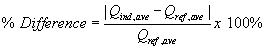(ii) To successfully pass the flow rate measurement accuracy test, the percent difference calculated in Equation 4 of this paragraph (g)(3) shall not exceed 2 percent.

(4) Flow rate coefficient of variation measurement accuracy. (i) Using the flow rate coefficient of variation indicated by the candidate test sampler at the completion of the 6-hour test (%CVind), determine the accuracy of this reported coefficient of variation as:

Equation 5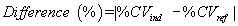(ii) To successfully pass the flow rate CV measurement accuracy test, the absolute difference in values calculated in Equation 5 of this paragraph (g)(4) must not exceed 0.3 (CV%).

(5) Flow rate cut-off. (i) Inspect the measurements of the sample flow rate during the flow rate cut-off test and determine the time at which the sample flow rate decreased to a value less than the cut-off value specified in table E-1 of this subpart. To pass this test, the sampler must have automatically stopped the sample flow at least 30 seconds but not more than 90 seconds after the time at which the sampler flow rate was determined to have decreased to a value less than the cut-off value.

(ii) At the completion of the flow rate cut-off test, download the archived data from the test sampler and verify that the sampler's required Flow-out-of-spec and Incorrect sample period flag indicators are properly set.

[62 FR 38799, July 18, 1997, as amended at 71 FR 61291, Oct. 17, 2006]